# Try These Mental Math Addition Strategies to Boost Math Fact Fluency

Math fact fluency is much more than simply speed and accuracy. True fluency also includes flexibility and appropriate strategy use. We can help students become flexible thinkers who are able to build their own understanding by reinforcing mental math addition strategies.

We know that being able to think flexibly is more effective than memorization for math facts. This has been studied in depth by many different math researchers. But sometimes what happens when we begin to teach strategies, is that we “teach” the strategies for memorization. For example, “Whenever you see numbers that differ by 1, you can use the doubles plus one strategy.” Rather than “teaching” the strategies, ideally we want students to discover them and construct their own understanding. The best way to do this is through lots and lots of work with manipulatives. And yes, even if you teach upper grades your students can benefit from manipulatives!

However, sometimes it’s still nice to have a guide for what strategies to steer our students toward.

## Seven Different Mental Math Addition Strategies

Below I have outlined seven different different mental math addition strategies that you can model in your classroom to help students build their understanding. I have also included videos and additional resources for some of them.

Counting On – Counting On is a beginning mental math strategy.  Counting on means that you start with the biggest number in an equation, and then count up. For example, in the equation 5+3, you want students to start with the “5” in their heads, and then count up, “6, 7, 8.” This is to discourage students from counting like, “1, 2, 3, 4, 5…..6, 7, 8.” Students also need to understand the commutative property of addition, where if an equation looks like this: “2+6,” they still should start with the bigger number (in this case, 6) and count up “7, 8.”

Here’s a video that will explain this strategy in more depth, or find a unit for teaching the counting on strategy HERE.

Doubles – The doubles are not necessarily a strategy, but rather a set of facts that is easy to learn and remember. Doubles are all around us; think of fingers and toes – 5+5, wheels on a car – 2+2, or the eggs in a carton – 6+6. Building a strong foundation of doubles will help students with the next strategy, Doubles Plus One.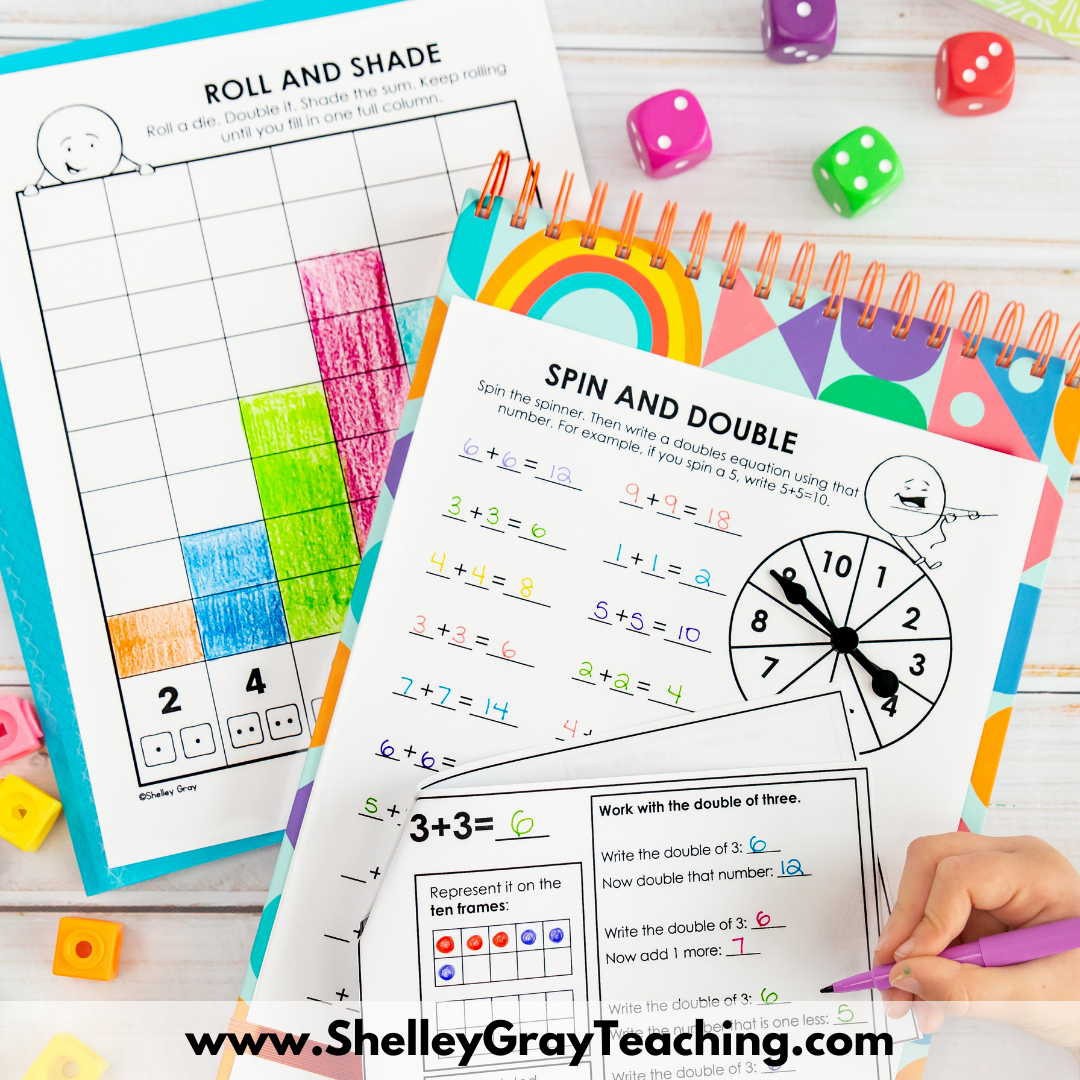Find a unit for teaching the doubles facts HERE.

Near Doubles – This strategy is a natural progression from the doubles. It includes using a known fact and building on it. For example, to solve 5+6, a student could think, “I know that 5+5 makes 10, and one more makes 11.” This strategy is best modelled with ten frames, which makes it so easy to see! And remember, we have to give our students the opportunity to SEE math so that they can build their understanding!

Here’s a video that explains the near doubles strategy in more depth or find a unit for teaching the near doubles facts HERE.

Make a Ten – Make a Ten is a mental math strategy where students use the number combinations that make ten to form connections and relationships to other facts. First, students must learn the number combinations that make 10. Then, they can confidently use those combinations. For example, to solve 8+5, a student might think, “I can take two from the 5 and give it to the 8 to make a ten, and then add the leftover 3 to make 13.” Ten frames are a fantastic way to illustrate this strategy.

Here’s a video that explains the make a ten strategy in more depth.

Find a unit for teaching make a ten HERE or find the math mats shown below HERE.Making Multiples of Ten – This strategy is a natural follow-up to making ten. To make multiples of ten, we can use the number combinations that make ten (6+4, 7+3, etc.). This helps us recognize that expressions such as 26+4 will make a multiple of ten. Ten frames are an excellent way to model the thinking process. For example, when we model 26+4 with a ten frame, it’s easy to see that we can shift the 4 dots over to completely fill three ten frames and make 30.

Find a unit for teaching the making multiples of ten strategy HERE.

Left to Right Addition – Left-to-right addition is a powerful mental math strategy for adding numbers with two or more digits. Place value understanding is key, as students will be grouping the tens and then the ones. For example, to solve 24+53, we will first add 20+50 to make 70, then 4+3 to make 7, and finally 70+7 to make 77. Left-to-right addition is important to teach BEFORE students learn the traditional algorithm. This is because left-to-right addition focuses on conceptual understanding rather than on the memorization of a series of steps.
Here’s a video that explains left to right addition in more depth.

Find a unit for teaching left to right addition HERE.

Break Apart/Decomposing – Breaking apart an addend by place value is a powerful mental math strategy for adding numbers with two or more digits. Although this is similar to left-to-right addition, some students prefer it because only one addend is decomposed by place value, rather than both of them. For example, to solve 43+35, we could first decompose the 35 into 30 and 5. We start by adding 43+30 to make 73, then the remaining 5 to make 78.

Here’s a video that explains the break apart strategy in more depth.

Find a unit for teaching break apart HERE.

I hope this post has helped you make a plan for teaching math strategies in your classroom! I know that if you did not learn this way, it is not an easy transition.

If you’d like more support for teaching addition strategies in the classroom, check out the Mental Math Addition Strategy Bundle that includes units for all of the strategies that were discussed above (photos of contents below).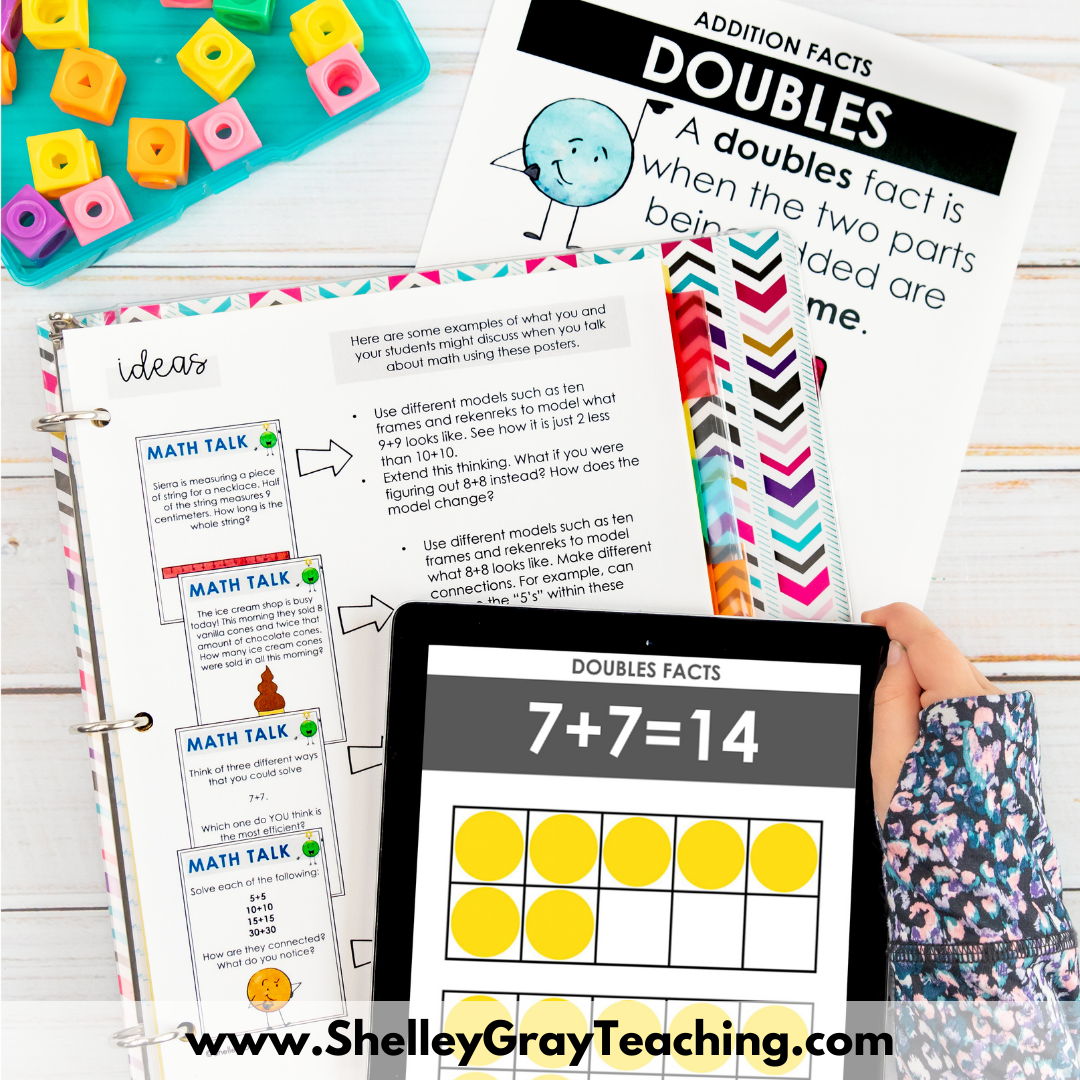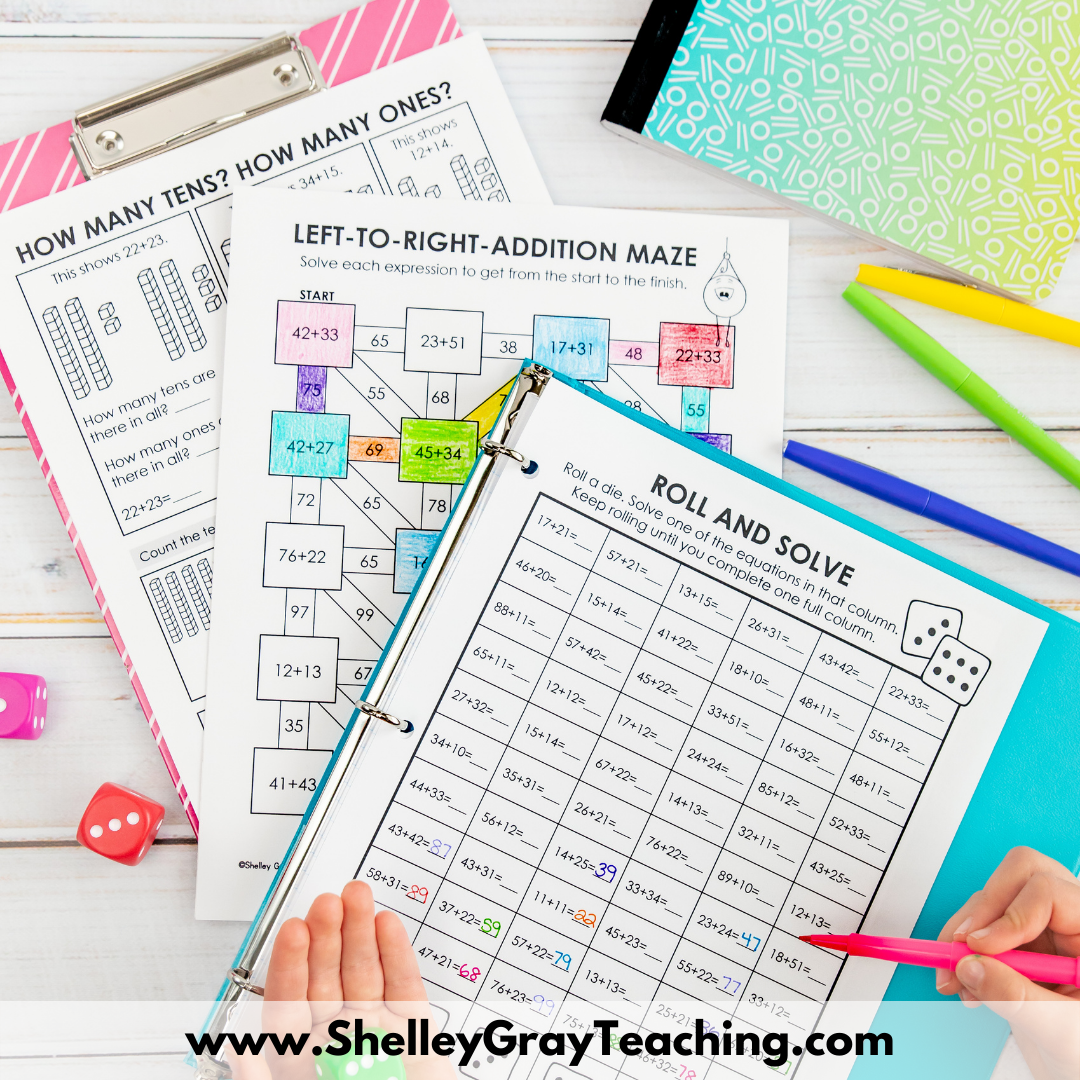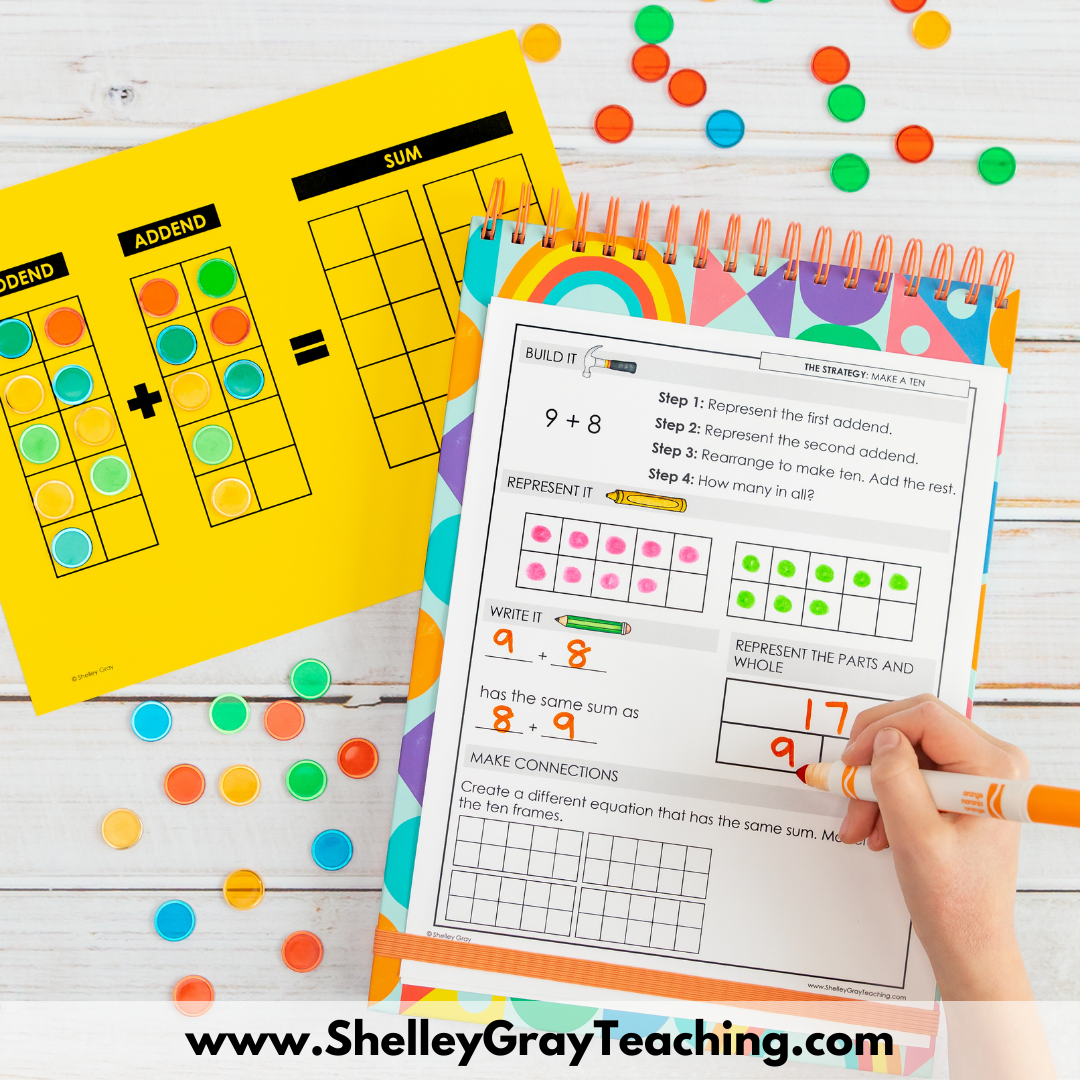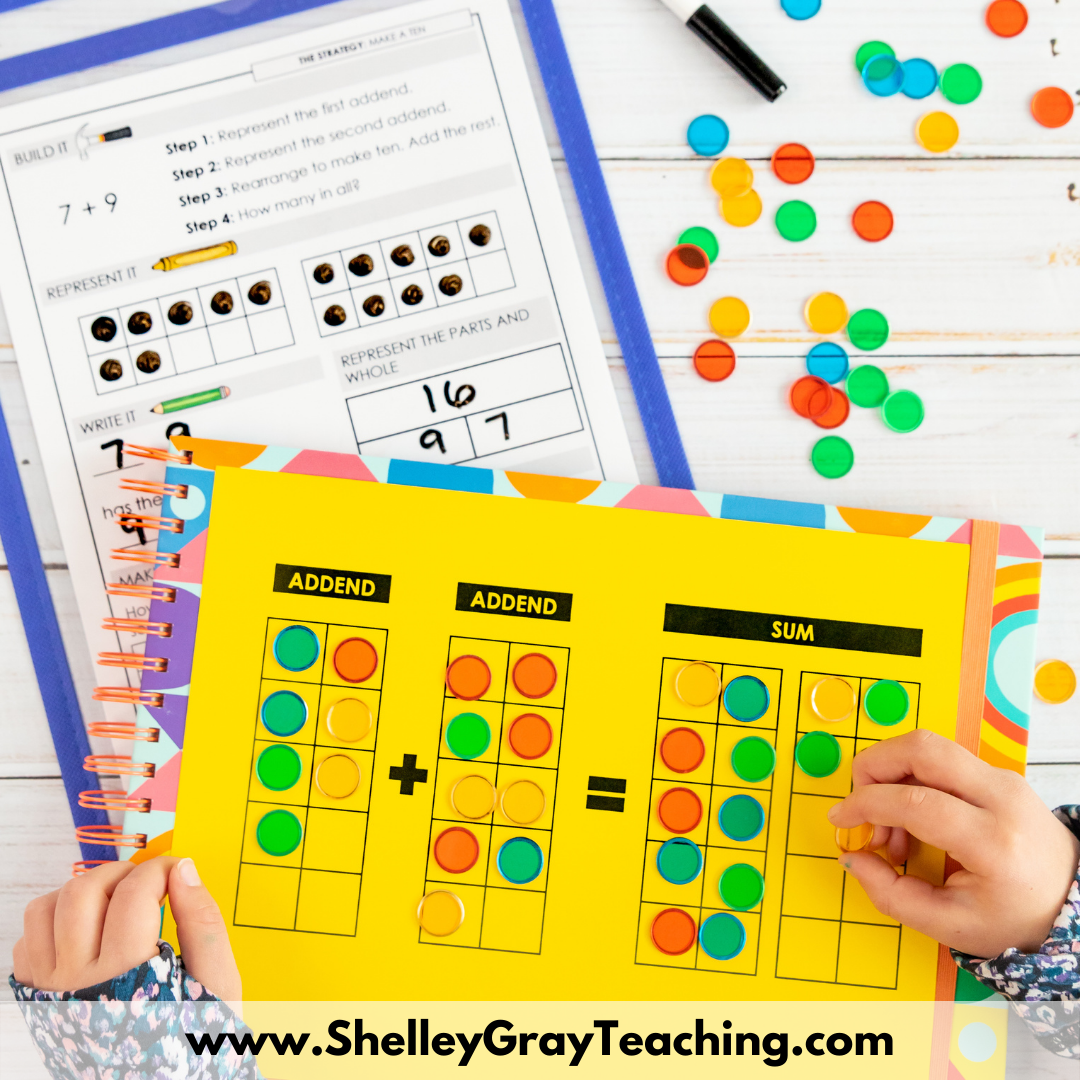•I love your blog and math strategies. I am trying to improve my math instruction this year and your strategies are great! Thanks for all the ideas.

•Hi…My little kid can do addition and subtraction but like you said he still goes back to counting.."1, 2, 3, etc.." Thanks for the counting on strategy, I will try this technique to advance his math skills… 🙂

•These strategies are great…they should also be taught to the parents, so parents can better support their children’s learning.

••Great! Love me some additional mental math.

•Lovely strategies. Good collection of all the mental strategies. Gonna try in my class.

•Thanks for sharing your work so everybody can learn from you. I celebrate innovation Keep up your hard.

••